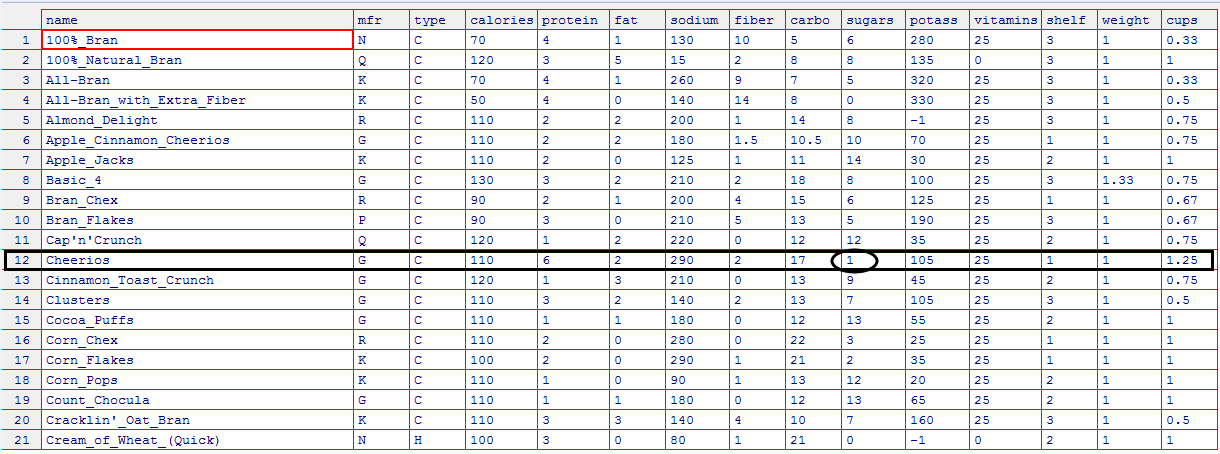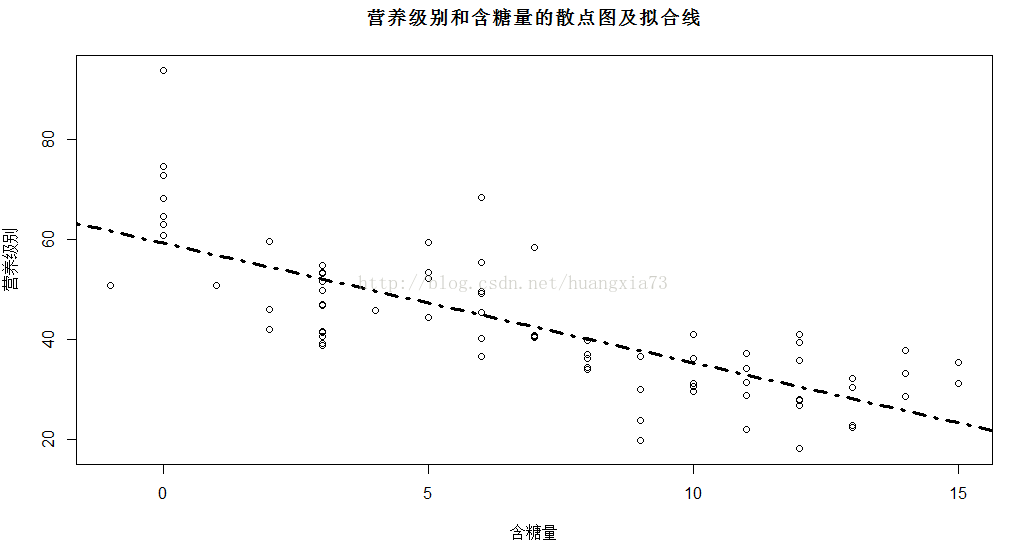# 数据挖掘方法之二：回归模型（简单线性回归）

## 一 概念

• $\bar y$是回应变量的估计值
• $b_0$是回归线在y轴上的截距
• $b_1$是回归线的斜率
• $b_0$和$b_1$称为回归系数

## 二 实例

    sugar<-read.table(file="/LabData/RData/regression/nutrition.txt",header=TRUE)


 edit（sugar）plot(data=sugar,rating~sugars,main="营养级别和含糖量的散点图及拟合线",xlab="含糖量",ylab="营养级别")

lm.reg<-lm(data=sugar,rating~sugars)

abline(lm.reg,lty=4,lwd=3)lm(data=sugar,rating~sugars)
Call:
lm(formula = rating ~ sugars, data = sugar)
Coefficients:
(Intercept)       sugars
59.284       -2.401


## 三 误差评估

### 1 最小二乘法估计

$y=m_0+m_1x+e$

1. 零均值假设：误差项是期望为零的随机变量，即$E(e)=0$
2. 不变方差假设：误差项e的方差（用$σ^2$表示）是常数且与 $x_1,x_2,….$ 的值无关
3. 独立性假设：e的变量是相互独立的
4. 正态性假设：误差项e是正态随机变量,也即：误差项e的值是独立的正态分布随机变量，带有均值0和不变方差$σ^2$

(1)根据零假设，回应变量y的值均落在回归线上 (2)根据不变方差假设，不论预测变量x1,x2,..取什么值，y的方差不变 (3)根据独立性假设，对任意的 $x_1,x_2,..$ 取值，y的值都是相互独立的 (4)根据正态性假设，回应变量y也是正态分布的随机变量。也即回应变量y也是独立正态变量，均值不变，方差不变。 最小二乘回归线(least-square line)将误差的平方和最小化，总的预测误差用SSEp表示，则总的误差平方和为：

$(y_i-\beta_0-\beta_1x_i)=0$

### 2 决定系数

$r^2$ 称为决定系数（coefficient of determination）用来衡量回归线的拟合度，也即最小二乘回归线产生的线性估计与实际观测数据的拟合程度。前面提到y^代表回应变量的估计值，$y-\bar y$ 代表预测误差或残差。

#### 引子

1.想象一下，如果不考虑数据集中含糖量而直接预测其营养价值，我们直观的做法是求其平均值作为预测值。假设开始为数据集里的每个记录计算(y-y’)（其中y’为回应变量的平均值），然后计算其平方和，这与计算误差(y-y^)，然后计算误差平方和类似。这时统计量总体误差平方和SST为：

SST，也称为总体平方和(sum of squares total，SST)是在没有考虑预测变量的情况下，衡量回应变量总体变异的统计量。

1. 接下来是衡量估计回归方程能多大程度提高估计的准确度。运用回归线时的估计误差为： $y-\bar y$ ,当忽略含糖量信息时，估计误差是 $y-y’$ 。因此改进量是：$\bar y-y’$ .进一步基于 $\bar y-y’$ 构造一个平方和的统计量，这样的统计量被称为回归平方和 (sum of squares of regression,SSR) ，是相对于忽略预测信息，衡量在使用回归线后预测精度提高的统计量，即：

#### 结论

SST衡量了回应变量变异的一部分，这部分是被回应变量和预测变量之间的线性关系所解释的。然而不是所有的数据点都正好落在回归线上，这意味着还有一部分y变量的变异不能被回归线所解释。SSE可以被认为是衡量不能被x和y之间的回归线所解释的其他变异，包括随机变异。 决定系数 $r^2$ ，它衡量了用回归线来描述预测变量和回应变量之间线性关系的符合程度

### 3 估计值的标准误差

S 值为“典型”残差的估计，s 是衡量估计中的典型误差，即回应预测值与实际值之间的差异。也即标准误差能反应估计回归方程做出预测的精确度，因此 s 越小越好。

### 4 其他评估

#### 1. 相关系数

• 其中 $S_x$和 $S_y$分别代表样本x和y的标准差
• 相关系数r的取值范围为：(-1,1)
• 变量r的值越接近于1，表明二者正向相关性越大，随着x增大y也会增大。
• 变量r的值越接近于-1，表明二者负向相关性越大，随着x增大y会减小。

#### 2. 方差分析表(ANOVA table)

anova<-aov(data=sugar,rating~sugars)
summary(anova)
Df Sum Sq Mean Sq  F value   Pr(>F)
sugars       1   8655    8655   102.3    1.15e-15
Residuals   75   6342      85

sugars      ***
Residuals
---
Signif. codes:  0 ‘***’ 0.001 ‘**’ 0.01 ‘*’ 0.05 ‘.’ 0.1 ‘ ’ 1



## 四 回归推断

1.用来推断回应变量与预测变量之间关系的t检验法

1. 斜率m1的置信区间
2. 在给定一个特定的预测值条件下，回应变量均值的置信区间
3. 在给定一个特定的预测值条件下，回应变量随机值的预测区间

### 4.1 x和y之间线性关系的t检验

#### 对斜率的估计

     lm.reg<-lm(data=sugar,rating~sugars)
summary(lm.reg)

Call:
lm(formula = rating ~ sugars, data = sugar)

Residuals:
Min      1Q  Median      3Q     Max
-17.853  -5.677  -1.439   5.160  34.421

Coefficients:
Estimate Std. Error t value Pr(>|t|)
(Intercept)  59.2844     1.9485   30.43  < 2e-16 ***
sugars       -2.4008     0.2373  -10.12 1.15e-15 ***
---
Signif. codes:  0 ‘***’ 0.001 ‘**’ 0.01 ‘*’ 0.05 ‘.’ 0.1 ‘ ’ 1

Residual standard error: 9.196 on 75 degrees of freedom
Multiple R-squared:  0.5771,    Adjusted R-squared:  0.5715
F-statistic: 102.3 on 1 and 75 DF,  p-value: 1.153e-15


• 在系数列下面，找到斜率m’的估计值为 -2.4008。
• 在SE系数列里找到斜率m’的标准差Sm’为 0.2373
• 在T值列找到t的统计量，即t检验的值 , $t=\frac{m’}{Sm’}=\frac{-2.4008}{0.2373}=-10.1171$
•  在最后一列可以找到t检验的p值，是一个双尾检验，形式为：p值= $P( t >tobs)$ ，其中的tobs代表观测值。此处P值= $P( t >tobs)=P( t >-10.1171)$ 近似为0，小于任何显著性要求的合理界限，因此可以拒绝零假设也即认为含糖量和营养级别之前存在线性关系。

### 4.2 回归直线斜率的置信区间

lm.reg<-lm(data=sugar,rating~sugars)
#level=0.95为置信度
confint(lm.reg,level=0.95)
2.5 %    97.5 %
(Intercept) 55.402783 63.165952
sugars      -2.873567 -1.92807


### 4.3 给定x条件下，y均值的置信区间和y随机选择值的预测区间

    > point<-data.frame(sugars=10)
> point
sugars
1     10
> lm.reg

Call:
lm(formula = rating ~ sugars, data = sugar)

Coefficients:
(Intercept)       sugars
59.284       -2.401

> lm.pred<-predict(lm.reg,point,interval="prediction",level=0.95)
> lm.pred
fit     lwr      upr
1 35.27617 16.7815 53.77083